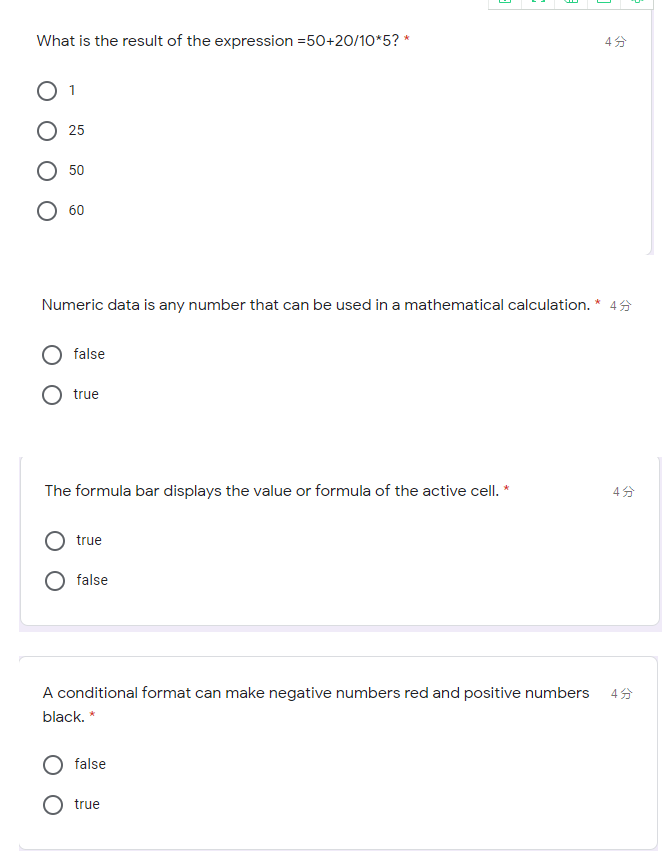# What is the result of the expression =50+20/10*5?What is the result of the expression =50+20/10*5?* 45 0 1 O 25 O 50 O 60 Numeric data is any number that can be used in a mathematical calculation. * 45 false O true The formula bar displays the value or formula of the active cell. * 45 true false A conditional format can make negative numbers red and positive numbers 4 black. false true

solution: 1)
=50+20/10*5
so, the given expression can be menually solved or solved in
excel based on the principal of BODMAS
so, in bodmas we first solve Brackets, Order or
power, Division, Multiplication, Addition and Substraction
so, 50+20/10*5
= 50+2*5 solved division
= 50+10 solved multiplication
so, correct answer is 60
solution2)
numeric data is any number that can be used in a mathemetical
calculation.
True,